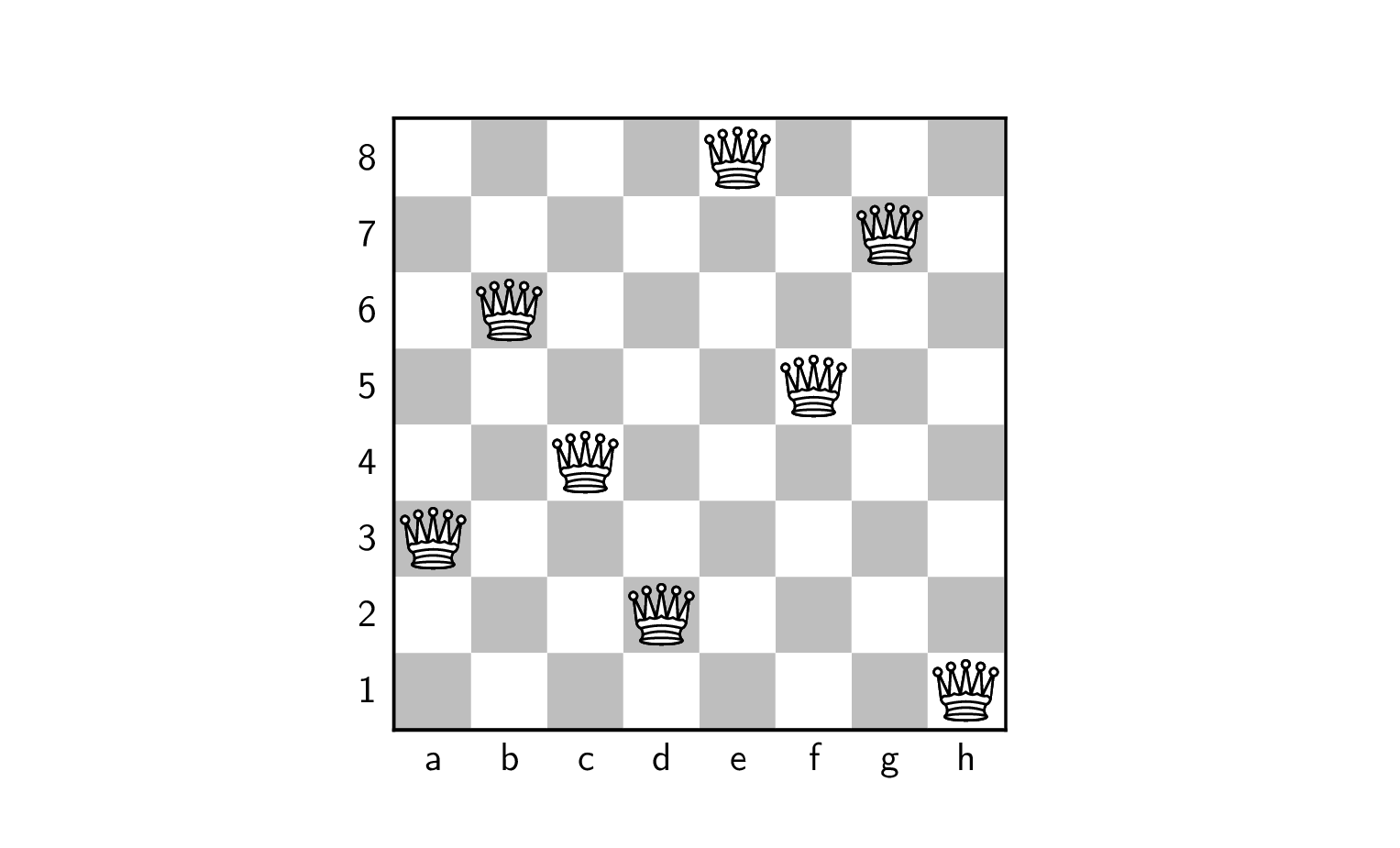PyCSP3

type: CSP
difficulty: easy

# Problem Queens

The problem is stated as follows: can we put 8 queens on a chessboard such that no two queens attack each other? Two queens attack each other iff they belong to the same row, the same column or the same diagonal.

By considering boards of various size, the problem can be generalized as follows: can we put $n$ queens on a board of size $n \times n$ such that no two queens attack each other? Contrary to previously introduced single problems, we have to deal here with a family of problem instances, each of them characterized by a specific value of $n$. We can try to solve the 8-queens instance, the 10-queens instance, and even the 1000-queens instance.

Here is a solution for the 8-queens problem instance:To build a CSP (Constraint Satisfaction Problem) model, we need first to import the library PyCSP$^3$:

from pycsp3 import *


Then, we need some data. Here, we just need to know the order (i.e., the number of queens). We choose 8.

n = 8


A simple model can be built by associating a variable with each row of the board. Thus, we start our CSP model with an array $q$ of $n$ variables, each one with ${0,1,\dots,n-1}$ as domain.

# q[i] is the column of the ith queen (at row i)
q = VarArray(size=n, dom=range(n))


Concerning the constraints, we can impose that queens are put on different columns by posting a constraint AllDifferent.

satisfy(
# all queens are put on different columns
AllDifferent(q)
);


Interestingly, by calling the function solve(), we can check that the problem is satisfiable (SAT). We can also display the found solution. Here, we call the function values() that collects the values assigned to a specified list of variables.

if solve() is SAT:
print(values(q))

[0, 1, 2, 3, 4, 5, 6, 7]


Of course, we have trivially a problem because all queens are put on the same diagonal. We can impose that no two queens are put on the same downward diagonal by adding this constraint (the proof is let as an exercice):

satisfy(
# no two queens on the same downward diagonal
AllDifferent(q[i] - i for i in range(n))
);


We can run the solver again:

if solve() is SAT:
print(values(q))

[0, 2, 6, 5, 7, 3, 1, 4]


We still have a problem with this “solution” because some queens are on the same upward diagonal. We can impose that no two queens are put on the same upward diagonal by adding this constraint (the proof is let as an exercice):

satisfy(
# no two queens on the same upward diagonal
AllDifferent(q[i] + i for i in range(n))
);


We can run the solver again:

if solve() is SAT:
print(values(q))

[5, 2, 0, 6, 4, 7, 1, 3]


This time, we do have a solution to the 8-queens problem instance. We can alos chack that there exist 92 solutions as follows:

if solve(sols=ALL) is SAT:
print("Number of solutions: ", n_solutions())

Number of solutions:  92


Finally, we give below the model in one piece. Here the data is expected to be given by the user (in a command line).

from pycsp3 import *

n = data

# q[i] is the column of the ith queen (at row i)
q = VarArray(size=n, dom=range(n))

satisfy(
# all queens are put on different columns
AllDifferent(q),

# no two queens on the same upward diagonal
AllDifferent(q[i] + i for i in range(n)),

# no two queens on the same downward diagonal
AllDifferent(q[i] - i for i in range(n))
)